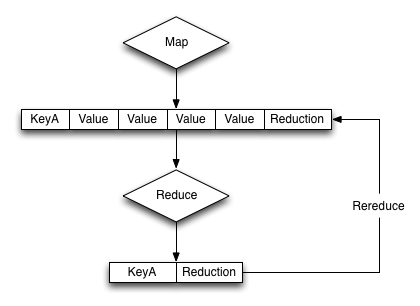A newer version of this documentation is available.

# Re-reduce Argument

+

For `reduce()` functions, they should be both transparent and standalone. For example, the `_sum` function did not rely on global variables or parsing of existing data, and didn’t need to call itself, hence it is also transparent.

In order to handle incremental map/reduce functionality (i.e. updating an existing view), each function must also be able to handle and consume the functions own output. This is because in an incremental situation, the function must be handle both the new records, and previously computed reductions.

This can be explicitly written as follows:

`f(keys, values) = f(keys, [ f(keys, values) ])`

The following diagram shows previous reductions included within the array of information that are re-supplied to the reduce function as an element of the array of values supplied to the reduce function.That is, the input of a reduce function can be not only the raw data from the map phase, but also the output of a previous reduce phase. This is called `rereduce`, and can be identified by the third argument to the `reduce()`. When the `rereduce` argument is true, both the `key` and `values` arguments are arrays, with the corresponding element in each containing the relevant key and value. I.e., `key` is the key related to the value of `value`.

An example of this can be seen by considering an expanded version of the `sum` function showing the supplied values for the first iteration of the view index building:

`function('James', [ 13000,20000,5000 ]) {...}`

When a document with the ‘James’ key is added to the database, and the view operation is called again to perform an incremental update, the equivalent call is:

`function('James', [ 19000, function('James', [ 13000,20000,5000 ]) ]) { ... }`

In reality, the incremental call is supplied the previously computed value, and the newly emitted value from the new document:

`function('James', [ 19000, 38000 ]) { ... }`

Fortunately, the simplicity of the structure for `sum` means that the function both expects an array of numbers, and returns a number, so these can easily be recombined.

If writing more complex reductions, where a compound key is output, the `reduce()` function must be able to handle processing an argument of the previous reduction as the compound value in addition to the data generated by the `map()` phase. For example, to generate a compound output showing both the total and count of values, a suitable `reduce()` function could be written like this:

```function(key, values, rereduce) {
var result = {total: 0, count: 0};
for(i=0; i < values.length; i++) {
if(rereduce) {
result.total = result.total + values[i].total;
result.count = result.count + values[i].count;
} else {
result.total = sum(values);
result.count = values.length;
}
}
return(result);
}```

Each element of the array supplied to the function is checked using the built-in `typeof` function to identify whether the element was an object (as output by a previous reduce), or a number (from the map phase), and then updates the return value accordingly.

Using the sample sales data, and group level of two, the output from a reduced view may look like this:

```{"rows":[
The `reduce()` function is designed to reduce and summarize the data emitted during the `map()` phase of the process. It should only be used to summarize the data, and not to transform the output information or concatenate the information into a single structure.
When using a composite structure, the size limit on the composite structure within the `reduce()` function is 64KB.